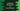# Introduction to JavaScript Arrow function## Definition :

Arrow functions are almost similar like the traditional JavaScript functions. You don’t need the function keyword and you need one arrow => between the arguments and function body. In this post, we will learn different ways to create an arrow function with different examples.

## Different types with different arguments :

The arrow function is much simpler. We can have shorter syntax with one or no parameter :

### Arrow function With one parameter :

Let’s consider one simple example :

``````const isEven = (x) => { return x % 2 == 0; }

console.log(isEven(3));
console.log(isEven(4));``````

Here, isEven is an arrow function that takes one parameter x and returns one boolean value based. true if x is even and false otherwise. For one statement arrow function that returns one value, we can remove the return keyword and the curly braces :

``````const isEven = (x) => x % 2 == 0;

console.log(isEven(3));
console.log(isEven(4));``````

It has only one argumet, for one argument arrow function, we can remove the parenthesis () :

``````const isEven = x => x % 2 == 0;

console.log(isEven(3));
console.log(isEven(4));``````

That looks much cleaner !

### Multi parameters :

For multiple parameters, you need the parenthesis. The return statement and curly braces are not required if it is one single statement :

``````const getProduct = (x,y) => x*y;

console.log(getProduct(3,4));``````

## No parameter :

If we don’t have any parameters, we can just use one empty parenthesis or one _. Both will work :

``````const getMessage = _ => "hello";

console.log(getMessage());``````

## Returning an object :

If you are returning one object, it will be slightly different :

``````const getMessage = _ => {message : "hello", count : 10}

console.log(getMessage());``````

It will not work because curly braces are used with a function body and if we want to return one object in single line statement, we need to put it inside parenthesis :

``````const getMessage = _ => ({message : "hello", count : 10})

console.log(getMessage());``````

## Multi line function :

Multi line arrow functions requires the body to be placed inside a curly braces :

``````const getMessage = loggedIn => {
if (loggedIn) {
return "Welcome back !!";
} else {
return "Welcome !!";
}
}

console.log(getMessage(true));
console.log(getMessage(false));``````

### this and arrow function :

For traditional functions, this represents the object which is calling it. No matter where it is defined. But for arrow functions, this represents the object where it is defined. We had few workarounds to bind this of current object before arrow function was introduced. Let me show you a couple of examples how it worked before and how it works now :

``````class MyClass {

constructor() {
this.myArr = [1, 2, 3, 4, 5];
this.multiplier = 2;
}

getNewArray() {
return this.myArr.map(function (x) {
return x * this.multiplier
})
}

}

let c = new MyClass();
console.log(c.getNewArray());``````

It will throw an error. You will get one TypeError :

``TypeError: Cannot read property 'multiplier' of undefined``

With map, we are using one normal function and this in that function is not represents the object of MyClass. We can use one reference variable to hold this.

``````class MyClass {

constructor() {
this.myArr = [1, 2, 3, 4, 5];
this.multiplier = 2;
}

getNewArray() {
var ref = this;
return this.myArr.map(function (x) {
return x * ref.multiplier
});
}

}

let c = new MyClass();
console.log(c.getNewArray());``````

Or, we can use bind :

``````class MyClass {

constructor() {
this.myArr = [1, 2, 3, 4, 5];
this.multiplier = 2;
}

getNewArray() {
return this.myArr.map(function (x) {
return x * this.multiplier
}.bind(this));
}

}

let c = new MyClass();
console.log(c.getNewArray());``````

Reference variable or bind works. But you don’t have to worry anything with an arrow function :

``````class MyClass {

constructor() {
this.myArr = [1, 2, 3, 4, 5];
this.multiplier = 2;
}

getNewArray() {
return this.myArr.map((x) => {
return x * this.multiplier
});
}

}

let c = new MyClass();
console.log(c.getNewArray());``````

It will print the modified array.

### Arrow functions with object functions :

``````const obj = {
message: "Hello World !!",
printMessage: () => this.message,
printMessageFunc: function () { return this.message }
}

console.log(obj.printMessage())
console.log(obj.printMessageFunc())``````

Arrow functions are not for method functions. The above example will print undefined for the first statement :

``````undefined
Hello World !!``````

It doesn’t work with object context.